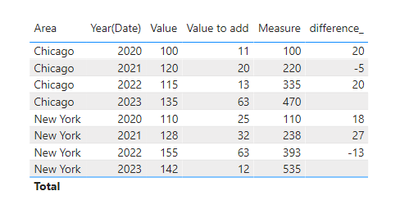cancel
Showing results for
Did you mean:Anonymous
Not applicable

## Incremental/Accumulative DAX/Query Calculation

Hi Power BI gurus!

I'm struggling with getting the incremental or accumulative formula over the years. Namely, my data has Area column, Date (Year) column, Value and Value to be Added. What I need is when I dynamically select years to show on my chart or table, use the first year's Value and then for each of the following years, keep adding the Value to Add to the previous calculation. This needs to be separate for each Area and not accumulated by Year. Below is the sample of the dataset.Appreciate any assistance! Thanks much!

1 ACCEPTED SOLUTIONSuper User

@Anonymous , A new column

new column =
var _min = minx(filter(Table, [Area] = earlier([Area]) ), Table[Year])
var _minval = sumx(filter(Table, [Area] = earlier([Area]) && Table[Year] =_min ), Table[Value])
return
sumx(filter(Table, [Area] = earlier([Area]) && Table[Year] >=_min && Table[Year] <= earlier(Table[Year]) ), Table[Value]) + _minval

A new measure

new measure =
var _min = minx(filter(allselected(Table), [Area] = max([Area]) ), Table[Year])
var _minval = sumx(filter(allselected(Table), [Area] = max([Area]) && Table[Year] =_min ), Table[Value])
return
sumx(filter(allselected(Table), [Area] = max([Area]) && Table[Year] >=_min && Table[Year] <= max(Table[Year]) ), Table[Value]) + _minval

3 REPLIES 3Community Support

Hi @Anonymous ,

Please refer to my pbix to see if it helps you.

Create measures.

``````Measure = CALCULATE(SUM('Table'[Value]),FILTER(ALL('Table'),'Table'[Year(Date)]<=SELECTEDVALUE('Table'[Year(Date)])&&'Table'[Area]=SELECTEDVALUE('Table'[Area])))
``````
``````difference_ =
VAR next_value =
CALCULATE (
MAX ( 'Table'[Value] ),
FILTER (
ALL ( 'Table' ),
'Table'[Area] = SELECTEDVALUE ( 'Table'[Area] )
&& 'Table'[Year(Date)]
= SELECTEDVALUE ( 'Table'[Year(Date)] ) + 1
)
)
VAR this_value =
MAX ( 'Table'[Value] )
VAR _a =
CALCULATE (
next_value - this_value,
FILTER ( ALL ( 'Table' ), 'Table'[Area] = SELECTEDVALUE ( 'Table'[Area] ) )
)
RETURN
IF ( next_value = BLANK (), BLANK (), _a )
``````Best Regards

Community Support Team _ Polly

If this post helps, then please consider Accept it as the solution to help the other members find it more quickly.Super User

@Anonymous , A new column

new column =
var _min = minx(filter(Table, [Area] = earlier([Area]) ), Table[Year])
var _minval = sumx(filter(Table, [Area] = earlier([Area]) && Table[Year] =_min ), Table[Value])
return
sumx(filter(Table, [Area] = earlier([Area]) && Table[Year] >=_min && Table[Year] <= earlier(Table[Year]) ), Table[Value]) + _minval

A new measure

new measure =
var _min = minx(filter(allselected(Table), [Area] = max([Area]) ), Table[Year])
var _minval = sumx(filter(allselected(Table), [Area] = max([Area]) && Table[Year] =_min ), Table[Value])
return
sumx(filter(allselected(Table), [Area] = max([Area]) && Table[Year] >=_min && Table[Year] <= max(Table[Year]) ), Table[Value]) + _minvalAnonymous
Not applicable

I got it to work with slight changes. Thanks much for the setup!

Btw, how would I summarize these calculations on a year level, and provide year-over-year variance of those sums?Announcements#### Exclusive opportunity for Women!

Join us for a free, hands-on Microsoft workshop led by women trainers for women where you will learn how to build a Dashboard in a Day!#### Power Platform Conference-Power BI and Fabric Sessions

Join us Oct 1 - 6 in Las Vegas for the Microsoft Power Platform Conference.Top Solution Authors
Top Kudoed Authors
Users online (2,090)